# Using sparseDFM - Inflation Example

Load the sparseDFM package and inflation dataframe into R:

library(sparseDFM)
data <- inflation 

This vignette provides a tutorial on how to apply the package sparseDFM onto a small subset of quarterly CPI (consumer price inflation) index data for the UK taken from the Office from National Statistics’ (ONS) website1. The data contains 36 variables of different classes of the inflation index and 135 observations from 1989 Q1 to 2022 Q32.

The purpose of this small, 36 variable example is to demonstrate the core functionality and the ability to graphically display results with the sparseDFM package. For a larger scale example, see the exports-example vignette, that aims to track monthly movements of UK Trade in Goods (exports) data using a high-dimensional set of indicators.

## Exploring the Data

Before we fit a model it is first worthwhile to perform some exploratory data analysis. Two main properties to look out for when working with dynamic factor models are the amount of missing data present in the data and if the data series are stationary or not. The function missing_data_plot() allows the user to visualise where missing data is present. The function transformData() allows the user to apply a stationary transformation to the data. It contains the parameter stationary_transform = options of: 1 for no change, 2 for first difference, 3 for second difference, 4 for log first difference, 5 for log second difference, 6 for growth rate and 7 for log growth rate.

# n = 135, p = 36
dim(data)
#>  135  36
# Names of inflation variables
colnames(data)
#>   "Vehicles Purchase (7.1)"            "Routine Maintenance (5.6)"
#>   "Tools and Equipment (5.5)"          "Glassware and Utensils (5.4)"
#>   "Household Appliances (5.3)"         "Household Textiles (5.2)"
#>   "Furniture and Carpets (5.1)"        "Fuels (4.5)"
#>   "Warer Supply (4.4)"                 "Repair of Dwelling (4.3)"
#>  "Actual Rents (4.1)"                 "Footwear (3.2)"
#>  "Clothing (3.1)"                     "Tobacco (2.2)"
#>  "Alcohol (2.1)"                      "Non-Alcoholic (1.2)"
#>  "Food (1.1)"                         "Peronal Care (12.1)"
#>  "Accomodation Services (11.2)"       "Catering Services (11.1)"
#>  "Recrational Services (9.4)"         "Other Recreational Items (9.3)"
#>  "Durables for Recreation (9.2)"      "AV Equipment (9.1)"
#>  "Fin Services NEC (12.6)"            "Post (8.1)"
#>  "Insurance (12.5)"                   "Transport (7.3)"
#>  "Social Protection (12.4)"           "Personal Transport Operation (7.2)"
#>  "Other Services (12.7)"              "Package Holidays (9.6)"
#>  "Books and Newspapers (9.5)"         "Hospital Services (6.3)"
#>  "Out Patient Services (6.2)"         "Medical Products (6.1)"
# Plot of data (standardised to mean 0 sd 1)
matplot(scale(data), type = 'l', ylab = 'Standardised Values', xlab = 'Observations')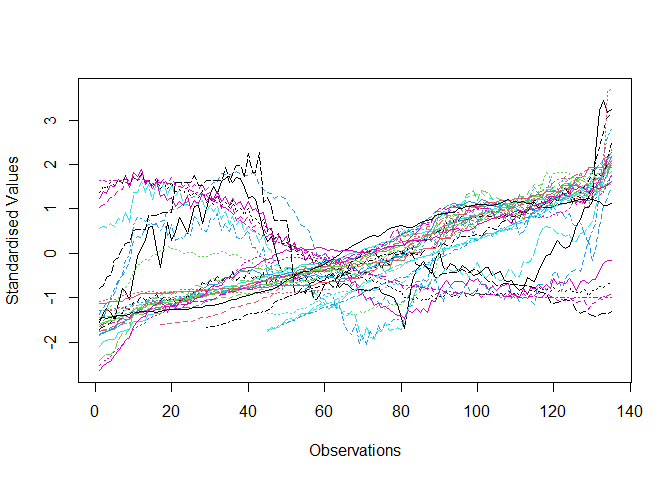The data does not look very stationary. Dynamic factor models (DFMs) assume the data is stationary and so therefore let’s try transforming the data by taking first differences.

# Take first differences
new_data = transformData(data, stationary_transform = rep(2,ncol(data)))
# Plot new_data (standardised to mean 0 sd 1)
matplot(scale(new_data), type = 'l', ylab = 'Standardised Values', xlab = 'Observations')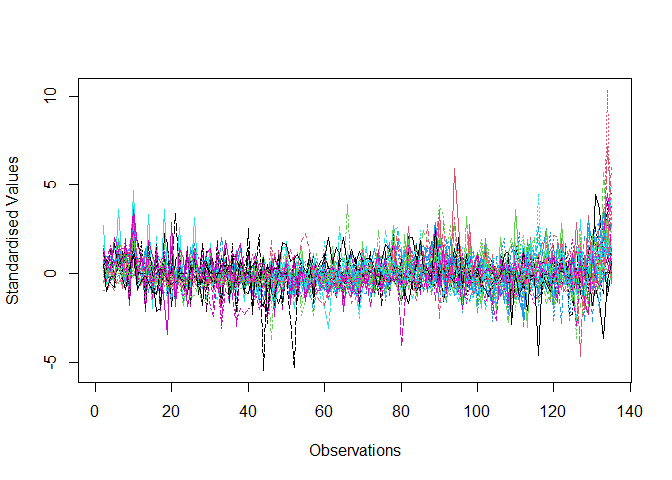The data now looks fairly stationary. Let’s now see if there is any missing data present.

missing_data_plot(data)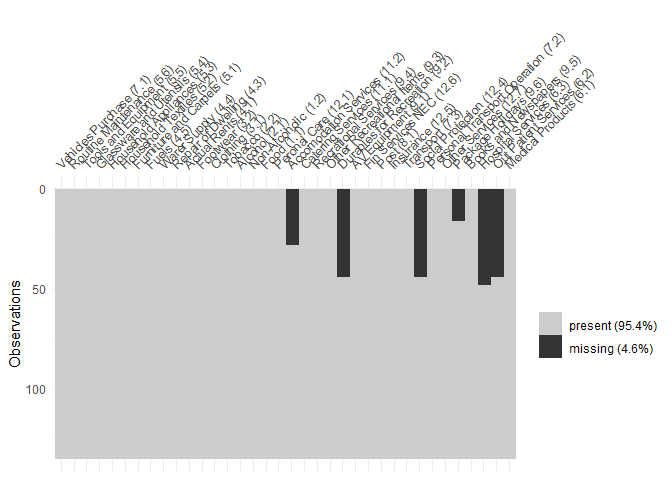We can see 6 variables have some missing data at the start of their sample. The state-space framework of DFMS allows them to handle arbitrary patterns of missing data present, so there is no need to worry. The package therefore may also be used as a practical way to perform missing data interpolation in large data sets.

## Structure of the Model

Before we fit a DFM, we first need to determine the best number of factors to use in the model. Our built-in function tuneFactors() uses the popular Bai and Ng (2002)3 information criteria approach to perform this tuning. The function prints out the optimal number of factors to use as chosen by information criteria type 1, 2 or 3 from Bai and Ng (2002) and also two plots. The first plot provides the information criteria value corresponding to the number of factors used and the second plot is a screeplot showing the percentage variance explained of the addition of more factors. In this type of plot we look for an ‘elbow’ in the curve to represent the point where the addition of more factors does not really explain much more of the variance in the data.

tuneFactors(new_data)
#> Data contains missing values: imputing data with fillNA()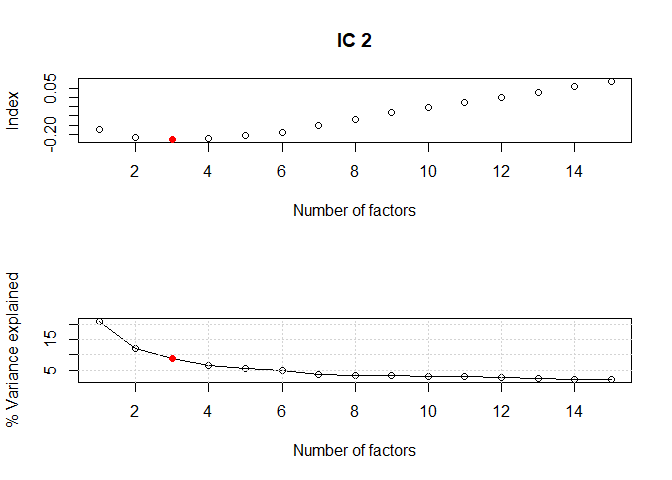#>  "The chosen number of factors using criteria type  2  is  3"

We find that the best number of factors to use for the (stationary) data is 3. We now can go ahead and fit a DFM and a Sparse DFM to the stationary data set to find factor estimates and explore the loadings matrix.

## Fitting a DFM

We begin by fitting a standard DFM to the data. This model follows the EM algorithm estimation approach of Banbura and Mudugno (2014)4, allowing for arbitrary patterns of missing data to be handled and efficient iterative estimation of model parameters using (quasi) maximum likelihood estimation. The standard DFM with 3 factors can be implemented like so:

fit.dfm <- sparseDFM(new_data, r=3, alg = 'EM', err = 'IID', kalman = 'univariate')
summary(fit.dfm)
#>
#> Call:
#>
#>  sparseDFM(X = new_data, r = 3, alg = "EM", err = "IID", kalman = "univariate")
#>
#>  Dynamic Factor Model using EM with:
#>
#>  n = 135 observations,
#>  p = 36 variables,
#>  r = 3 factors,
#>  err = IID
#>
#>  The r x r factor transition matrix A
#>            F1         F2          F3
#> F1  0.3471944  0.8672512 -0.69581098
#> F2  0.5341667 -0.3907927 -0.39801816
#> F3 -0.2009368  0.4740057  0.08717941
#>
#>
#>  The r x r factor transition error covariance matrix Sigma_u
#>            u1         u2         u3
#> u1  3.5334868 -0.2695235 -0.5345583
#> u2 -0.2695235  2.4666091  0.7245212
#> u3 -0.5345583  0.7245212  2.5614458

We set alg = 'EM' for the DFM estimation technique of Banbura and Mudogno (2014). Alternatives here would be to set alg = 'PCA' for a principle components analysis solution as in Stock and Watson (2002)5. Or alg = '2Stage' for the two-stage PCA + Kalman smoother estimation from Giannone et al. (2008)6. Or alg = 'EM-sparse' for the new Sparse DFM technique of (cite) to induce sparse loadings. For the EM algorithm, we use the default settings of max_iter = 100 and threshold = 1e-04 for the maximum number of iterations of the EM algorithm and the convergence threshold respectively. This can be changed.

We set err = 'IID' for IID idiosyncratic errors and kalman = 'univariate' for an implementation of the fast univariate filtering technique for kalman filter and smoother equations of Koopman and Durbin (2000)7. Note, we could set err = 'ar1' for idiosyncratic errors following an AR(1) process, however, this comes at a cost of being slower than the IID error case as the AR(1) errors are added as latent states to the model. It is better to use kalman = 'multivariate' when using alg = 'ar1' as univariate filtering a lot of states is costly.

The package sparseDFM provides many useful S3 class plots to visualise the estimated factors and loadings and provide information on the EM algorithm.

# Plot all of the estimated factors
plot(fit.dfm, type = 'factor')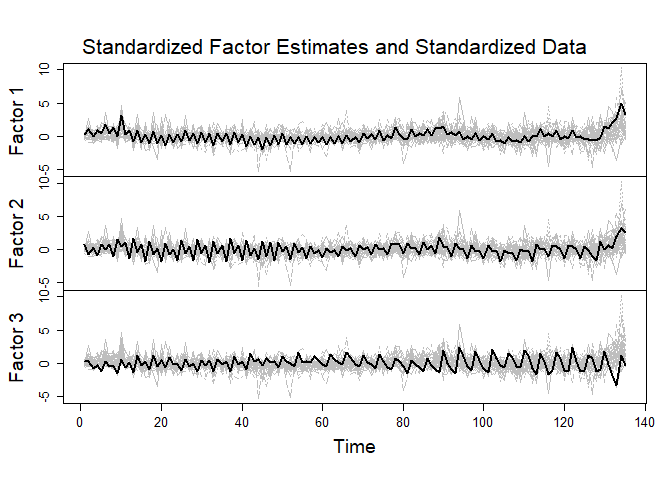We can see that 3 factors (solid black lines) are capturing the majority of the variance of the stationary data (grey). The user has options to change the number of factors to display by setting the parameter which.factors to be equal to a vector between 1 and $$r$$ (the number of factors). Also, able to change the colours by using parameters series.col and factor.col.

# Plot a heatmap of the loadings for all factors
plot(fit.dfm, type = 'loading.heatmap', use.series.names = TRUE)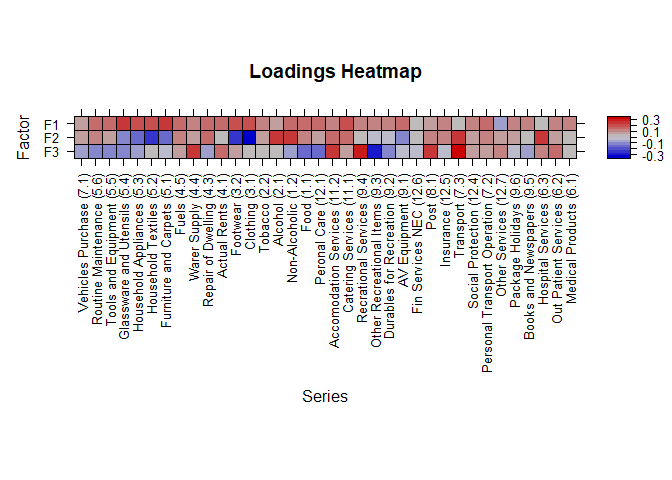The loadings heatmap plot is really useful to see the weight each variable has on the factors. If the data matrix has variable names then the plot will display these names. Set use.series.names to FALSE for numbers. You are able to specify which series to show using parameter which.series. The default is all parameters, 1:$$p$$. Similarly, choose which factors to show with which.factors.

# Plot a line plot for the loadings for factor 1
plot(fit.dfm, type = 'loading.lineplot', loading.factor = 1, use.series.names = TRUE)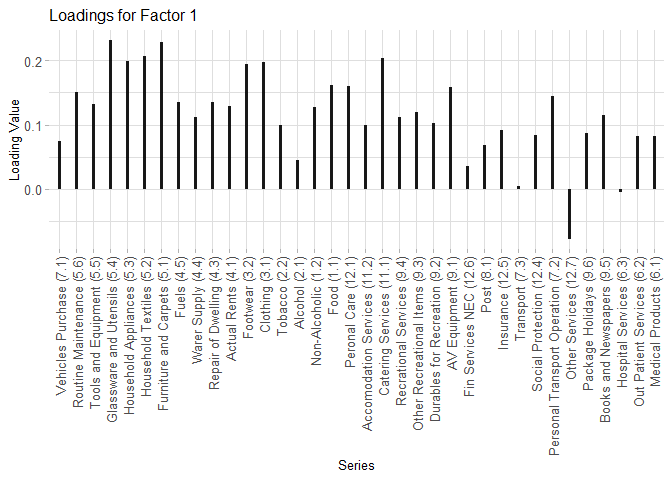The loading lineplot is an alternative way of displaying the contribution of the variables to the factors. Choose which factor to display using loading.factor.

# Plot boxplots for the residuals of each variable
plot(fit.dfm, type = 'residual', use.series.names = TRUE)
#> Warning in FUN(newX[, i], ...): NAs introduced by coercion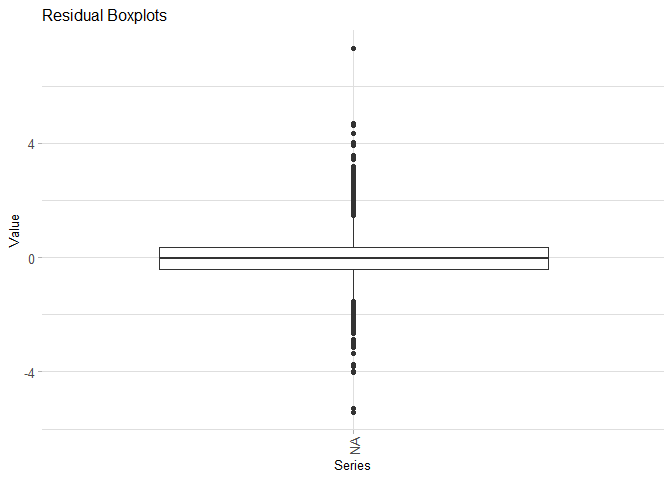To make a plot of the residuals for each variable set type = 'residual'. For a scatterplot of a particular variable add the parameters residual.type = 'scatter' and scatter.series = 1 or whichever variable series you wish to plot.

It may also be informative to look into the convergence of the EM algorithm. Possible ways of doing this are like so:

# Did the EM algorithm converge?
fit.dfm$em$converged
#>  TRUE
# How many iterations did the EM take to converge?
fit.dfm$em$num_iter
#>  17
# What were the log-likelihood values at each EM iteration?
fit.dfm$em$loglik
#>   -5551.698 -5521.428 -5514.720 -5511.251 -5508.877 -5507.059 -5505.556
#>   -5504.268 -5503.140 -5502.136 -5501.235 -5500.419 -5499.677 -5498.998
#>  -5498.375 -5497.801 -5497.271
# Plot these log-likelihood values
plot(fit.dfm, type = 'em.convergence')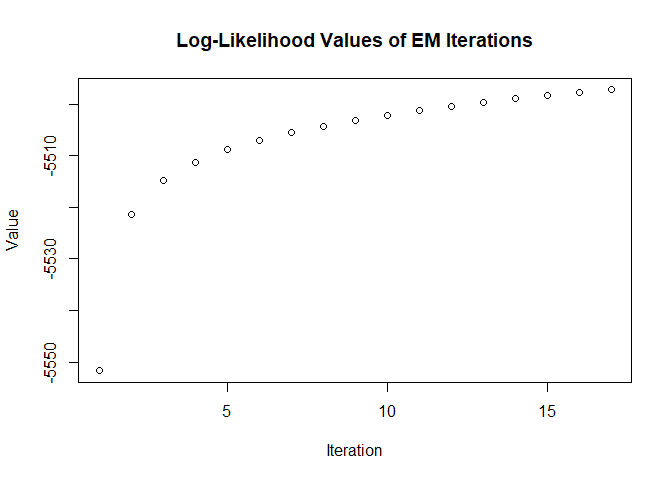Finally, we can use the DFM fit to return fitted values for dataset giving us predictions for any missing data present. This can be found by calling fit.dfm\$data\$fitted.unscaled (or fit.dfm\$data\$fitted for the standardised fit). Also, we can forecast future values of the data and of the festimated factors by using predict() like so:

# Forecast 3 steps ahead of the sample
my_forecast <- predict(fit.dfm, h = 3)
my_forecast
#>
#>  The 3 step ahead forecast for the data series are
#>           [,1]     [,2]     [,3]     [,4]     [,5]      [,6]     [,7]      [,8]
#> [1,] 1.6141662 1.805471 1.853779 2.524073 2.138883 1.7938710 2.873018 11.191750
#> [2,] 1.2849043 1.463686 1.430449 1.533842 1.114832 0.4584415 1.624292  7.992851
#> [3,] 0.9690882 1.213461 1.118131 1.445429 1.201490 0.9216629 1.781161  6.537968
#>          [,9]    [,10]    [,11]     [,12]      [,13]    [,14]    [,15]    [,16]
#> [1,] 2.967309 1.585391 1.467134 2.6384972  3.3877380 2.095370 2.159940 2.630586
#> [2,] 1.955870 1.319944 1.060719 0.1940032 -0.4186159 1.701435 1.881253 2.194750
#> [3,] 1.905407 1.096929 1.060378 1.2414753  1.5356735 1.565297 1.321266 1.641222
#>         [,17]     [,18]    [,19]    [,20]    [,21]     [,22]     [,23]
#> [1,] 2.361959 1.4946260 3.609590 1.989242 2.030794 0.9573333 1.0912529
#> [2,] 1.878070 1.1995203 2.574275 1.563397 1.352476 0.7923386 0.9112358
#> [3,] 1.493236 0.9991743 2.315533 1.379486 1.404355 0.5732896 0.8429813
#>          [,24]     [,25]    [,26]    [,27]    [,28]    [,29]    [,30]     [,31]
#> [1,]  1.587319 1.3064817 2.788295 2.726155 4.358169 1.159933 4.506793 0.1435845
#> [2,] -1.435455 0.8479793 1.957864 2.215294 3.333907 1.058105 3.293578 0.2831045
#> [3,] -1.310539 0.6668378 1.861091 1.833899 2.754783 1.019818 2.801144 0.3003135
#>         [,32]    [,33]    [,34]     [,35]     [,36]
#> [1,] 1.632103 1.603435 2.211268 1.2456248 1.0485072
#> [2,] 1.351200 1.317804 2.078259 1.0031254 0.8217618
#> [3,] 1.189950 1.178027 1.674637 0.9571129 0.7489041
#>
#>  The 3 step ahead forecast for the factors are
#>          [,1]     [,2]       [,3]
#> [1,] 9.507421 3.527408  0.7365877
#> [2,] 5.847546 3.406887 -0.1741638
#> [3,] 5.106048 1.861498  0.4247134

## Fitting a Sparse DFM

Now let’s see how we can fit a Sparse DFM to the inflation data.

fit.sdfm <- sparseDFM(new_data, r = 3, alphas = logspace(-2,3,100), alg = 'EM-sparse', err = 'IID', kalman = 'univariate')
summary(fit.sdfm)
#>
#> Call:
#>
#>  sparseDFM(X = new_data, r = 3, alphas = logspace(-2, 3, 100),      alg = "EM-sparse", err = "IID", kalman = "univariate")
#>
#>  Sparse Dynamic Factor Model using EM-sparse with:
#>
#>  n = 135 observations,
#>  p = 36 variables,
#>  r = 3 factors,
#>  err = IID
#>
#>  The r x r factor transition matrix A
#>            F1         F2          F3
#> F1  0.4654857  0.9849589 -0.96021652
#> F2  0.6433499 -0.3851340 -0.55318409
#> F3 -0.2577013  0.9030837 -0.02329362
#>
#>
#>  The r x r factor transition error covariance matrix Sigma_u
#>            u1         u2         u3
#> u1  3.5334868 -0.2695235 -0.5345583
#> u2 -0.2695235  2.4666091  0.7245212
#> u3 -0.5345583  0.7245212  2.5614458

We tune for the best $$\ell_1$$-norm penalty parameter using BIC8 across a logspace grid of values from $$10^{-2}$$ to $$10^{3}$$. This is the default grid to cover a wide range of values. The user can input any grid or a single value for the penalty using parameter alphas =. We stop searching over the grid of alphas as soon as an entire column of the loadings matrix $${\Lambda}$$ is entirely 0. We can find which $$\ell_1$$-norm penalty parameter was chosen as best and observe the BIC values for each parameter considered by doing:

# Grid of alpha values used before a column of Lambda was set entirely to 0
fit.sdfm$em$alpha_grid
#>    0.01000000  0.01123324  0.01261857  0.01417474  0.01592283  0.01788650
#>    0.02009233  0.02257020  0.02535364  0.02848036  0.03199267  0.03593814
#>   0.04037017  0.04534879  0.05094138  0.05722368  0.06428073  0.07220809
#>   0.08111308  0.09111628  0.10235310  0.11497570  0.12915497  0.14508288
#>   0.16297508  0.18307383  0.20565123  0.23101297  0.25950242  0.29150531
#>   0.32745492  0.36783798  0.41320124  0.46415888  0.52140083  0.58570208
#>   0.65793322  0.73907220  0.83021757  0.93260335  1.04761575  1.17681195
#>   1.32194115  1.48496826  1.66810054  1.87381742  2.10490414  2.36448941
#>   2.65608778  2.98364724  3.35160265  3.76493581  4.22924287  4.75081016
#>   5.33669923  5.99484250  6.73415066  7.56463328  8.49753436  9.54548457
#>  10.72267222 12.04503540 13.53047775 15.19911083
# The best alpha chosen
fit.sdfm$em$alpha_opt
#>  0.4641589
# Plot the BIC values for each alpha
plot(fit.sdfm, type = 'lasso.bic')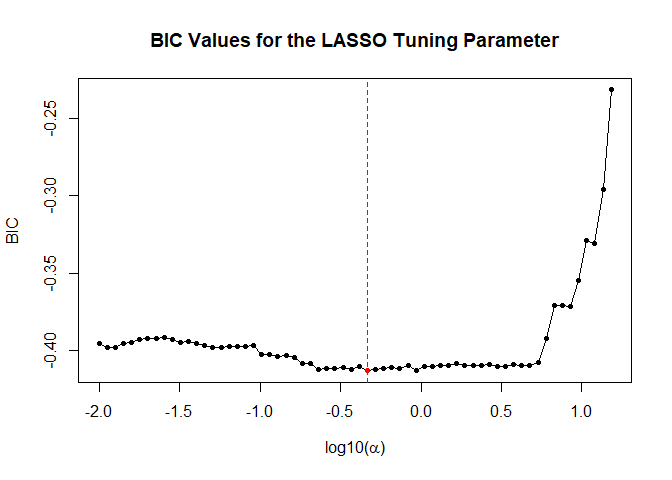Let’s view a heatmap of the estimated loadings to see if any variables have become sparse:

plot(fit.sdfm, type = 'loading.heatmap', use.series.names = TRUE)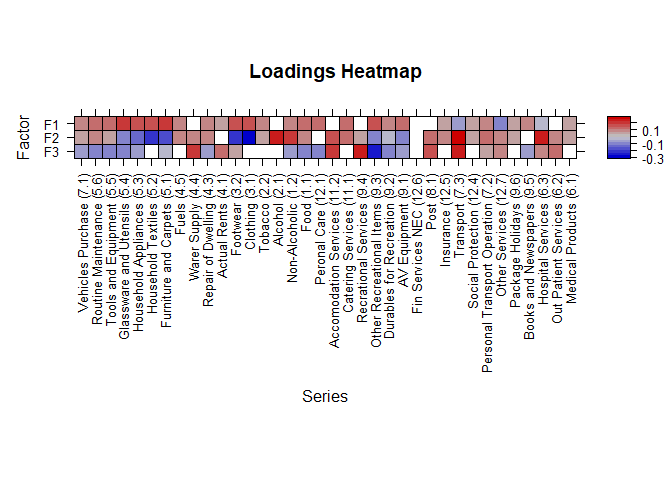It is easily to visualise sparsity in this heatmap as white grids represent 0 loading values. We find in some factors, certain variables are set to 0. This provides a bit more interpretation into how the inflation data are loaded onto the estimated factors of the model.

1. The data is from the Q4 2022 release and is benchmarked to 2015=100↩︎

2. Bai, J., & Ng, S. (2002). Determining the number of factors in approximate factor models. Econometrica, 70(1), 191-221.↩︎

3. Bańbura, M., & Modugno, M. (2014). Maximum likelihood estimation of factor models on datasets with arbitrary pattern of missing data. Journal of applied econometrics, 29(1), 133-160.↩︎

4. Stock, J. H., & Watson, M. W. (2002). Forecasting using principal components from a large number of predictors. Journal of the American statistical association, 97(460), 1167-1179.↩︎

5. Giannone, D., Reichlin, L., & Small, D. (2008). Nowcasting: The real-time informational content of macroeconomic data. Journal of monetary economics, 55(4), 665-676.↩︎

6. Koopman, S. J., & Durbin, J. (2000). Fast filtering and smoothing for multivariate state space models. Journal of time series analysis, 21(3), 281-296.↩︎

7. See (cite)↩︎